StatLect

# Ensembling

In machine learning, the best predictive performance is often obtained by averaging the forecasts from different models, a process which is called ensembling or ensemble learning.

Warning: ensembling is a vast topic and we are going to only scratch the surface here.## Theory

Suppose that we have trained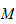different predictive modelsThe set ofmodels is called an ensemble.

The ensemble average is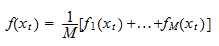It is possible to prove (e.g., Sollich and Krogh 1996) thatNote that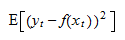is the mean squared error (MSE) of the ensemble average andis the MSE of a single predictive model.

The last term, that is,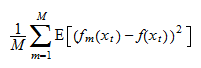is a measure of the diversity (or disagreement) among the models.

Therefore, the MSE of the ensemble average is less than the average MSE of the models in the ensemble. How much less? It depends on the diversity of the ensemble. The more diverse the ensemble, the greater the reduction in MSE.

Remarks:

• we have used a simple average of predictive models above, but the same result holds if we use weighted averages (different models are assigned different weights);

• from an empirical viewpoint, the result proved above for the squared error generally applies also to other loss functions. In other words, when building predictive models, we usually find that the estimated risk of the ensemble average is equal to the average risk of the single models less a quantity that is increasing in model diversity.

## Practice

How to exploit the above theoretical result in practice is more of an art than a science.

There is a vast literature on ensembling which we cannot cover in this introductory course.

Here, we provide a simple recipe that can be applied in most scenarios.

Suppose that we have decided to use a certain algorithm (e.g., boosted trees, as implemented in LightGBM). Then, it is basically a free lunch to use the same algorithm to train different models by randomizing along the following dimensions:

• keep the test sample fixed, and create several different random partitions of the remaining observations into training and validation samples;

• randomly pick the values of some hyper-parameters of the algorithm (e.g., the learning rate, the maximum depth of the decision trees) from sets of values that we deem equally reasonable;

• randomly drop a small portion of the inputs (so-called feature bagging).

In the next lecture, we will also see how to create ensembles by using a smart form of cross-validation called K-fold cross-validation.

## Python example

For this example, we use the same artificially-generated data set used in the lecture on boosted trees:

• there are 300 correlated variables in the input vector;

• the output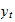is a function of only 10 of them;

• the 10 relevant inputs have:

• linear effects;

• non-linear effects (square, log, cos);

• interaction effects (products);

• threshold effects (some are relevant only if others are above threshold);

• there are 500 observations in the data set.

### Import the data and use scikit-learn to split into train_val-and-test (80-20)

We import the data and split it into train_val and test.

Subsequently, train_val will be split randomly into train and val in a different manner for each model in the ensemble.

Note that the split is done in such a way that the test set is identical to that used in previous lectures.

``````# Import the packages used to load and manipulate the data
import numpy as np # Numpy is a Matlab-like package for array manipulation and linear algebra
import pandas as pd # Pandas is a data-analysis and table-manipulation tool

# Import the function that performs sample splits from scikit-learn
from sklearn.model_selection import train_test_split

try:
except:
y = y.values # Transform y into a numpy array

# Print some information about the output variable
print('Class and dimension of output variable:')
print(type(y))
print(y.shape)

# Load the input variables with pandas
try:
except:
x = x.values

# Print some information about the input variables
print('Class and dimension of input variables:')
print(type(x))
print(x.shape)

# The code below is ugly! Done to have same test set as in previous lectures
x_train, x_val_test, y_train, y_val_test
= train_test_split(x, y, test_size=0.4, random_state=0)

x_val, x_test, y_val, y_test
= train_test_split(x_val_test, y_val_test, test_size=0.5, random_state=0)
y_test = np.squeeze(y_test)

x_train_val = np.vstack((x_train, x_val))
y_train_val = np.vstack((y_train, y_val))

# Print the numerosities of the three samples
print('Numerosities of training, validation and test samples:')
print(x_train.shape, x_val.shape, x_test.shape)``````

The output is:

``````Class and dimension of output variable:
class 'numpy.ndarray'
(500, 1)
Class and dimension of input variables:
class 'numpy.ndarray'
(500, 300)
Numerosities of training, validation and test samples:
300 100 100``````

### Create an ensemble of 100 models with LightGBM

Our ensemble comprises 100 different models.

Differences among models are generated by:

• different random splits into train an validation sets;

• random choices of the following hyper-parameters:

• learning rate;

• maximum depth of the trees;

• minimum number of observations in a leaf;

• number of early stopping rounds (i.e., maximum tolerated number of iterations without an improvement in the validation loss);

• randomly dropping 20 per cent of the input variables at each iteration of the boosting algorithm.

``````#Import the lightGBM package
import lightgbm as lgb

# Import model-evaluation metrics
from sklearn.metrics import mean_squared_error, r2_score

# Import random number generator and set seed
import random
random.seed(10)

# Set number of models in the ensemble and model list
n_models = 100
ensemble = []

for j in range(n_models):
# Randomly partition the train_val set
x_train, x_val, y_train, y_val
= train_test_split(x_train_val, y_train_val, test_size=0.25, random_state=j)

# Prepare dataset in LightGMB format
y_train = np.squeeze(y_train)
y_val = np.squeeze(y_val)
train_set = lgb.Dataset(x_train, y_train, silent=True)
valid_set = lgb.Dataset(x_val, y_val, silent=True)

# Randomly choose hyperparameter values
learning_rate = random.choice([0.05, 0.075, 0.10, 0.125, 0.15])
max_depth = random.choice([2, 3])
min_data_in_leaf = random.choice([5, 10, 15])
early_stopping_rounds = random.choice([15, 20, 25])

# Set algorithm parameters
params = {
'objective': 'regression',
'learning_rate': learning_rate,
'metric': 'mse',
'min_data_in_leaf': min_data_in_leaf,
'max_depth': max_depth,
'seed': j,
'feature_fraction': 0.8,
'verbose': -1
} # The feature_fraction parameter allows us to randomize over inputs

# Train the model
boosted_tree = lgb.train(
params = params,
train_set = train_set,
valid_sets = valid_set,
num_boost_round = 10000,
early_stopping_rounds =  early_stopping_rounds,
verbose_eval = False,
)

# Save the model in the ensemble list
ensemble.append(boosted_tree)

# Compute ensemble average and MSEs of single models
mses_single_models = []
y_test_pred_ensemble_avg = 0
for j in range(n_models):
y_test_pred = ensemble[j].predict(x_test)
y_test_pred_ensemble_avg += y_test_pred / n_models
mse = mean_squared_error(y_test, y_test_pred)
mses_single_models.append(mse)

# Compute average MSE of models and MSE of ensemble average on test set
print('Average test MSE of models in the ensemble:')
print(np.mean(mses_single_models))
print('Test MSE of ensemble average:')
print(mean_squared_error(y_test, y_test_pred_ensemble_avg))
print('')

# Print R squared on test set
print('R squared of ensemble average on test set:')
print(r2_score(y_test, y_test_pred_ensemble_avg))``````

The output is:

``````Average test MSE of models in the ensemble:
56.17079946910855
Test MSE of ensemble average:
50.204065172974545

R squared of ensemble average on test set:
0.7609487489387105``````

The test MSE of the ensemble average is significantly lower than the average MSE of the models in the ensemble. By generating an ensemble, with little effort we achieve an average reduction in test MSE larger than 10 per cent.

## References

Sollich, P. and Krogh, A. (1996) "Learning with ensembles: How overfitting can be useful," Advances in Neural Information Processing Systems, volume 8, pp. 190-196.